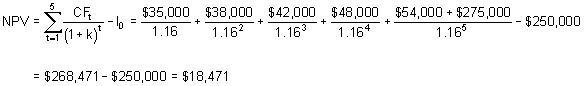### CFA Practice Question

There are 195 practice questions for this study session.

### CFA Practice Question

An investor is considering building a small office park that she plans to hold for five years before selling. The investor's initial outlay of \$250,000 would yield after-tax cash flows in years one through five of \$35,000, \$38,000, \$42,000, \$48,000, and \$54,000, respectively. It is anticipated that the office park will be sold at the end of the fifth year for net after-tax proceeds of \$275,000. If the investor has a required rate of return of 16%, the net present value of this real estate investment would be closest to:
A. -\$14,773.
B. \$18,471
C. \$27,899
Explanation: The net present value would be computed as:User Comment
Bibhu The way to calculate in the BA II plus as follows.
1. CF
2. 2nd + clear work.
3.250000 +/- Enter ( CF0= -250000)
4. C01= 35000, F01=1
C02= 38000, F02 =1
C03= 42000, F03 =1
C04= 48000, F04 =1
C05= 54000+27500 F05=1
5.CPT + NPV
6. I =16
7.down arrow
8. CPT NPV = 18471
kellyyang Saving time !